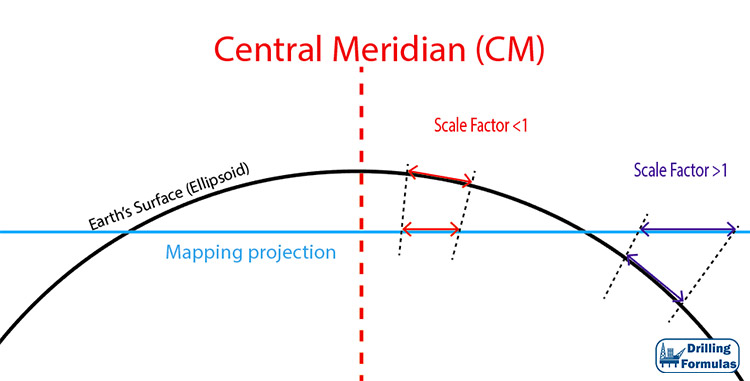## Scale Factor in UTM Mapping System and its Application in Directional DrillingScale factor represents distortion from a mapping system since the Earth is mapped into a flat surface, but the actual surface is in curvature. Figure 1 illustrates a scale factor with a reference location of the Earth’s surface. If the location is above the map projection plan, the scale factor will be less than 1.0. However, if the location is below the mapping projection, the scale factor will be more than 1.0. A scale factor less than 1 means that the actual distance on the Earth’s surface is longer than the actual distance on the map. Whereas, the scale factor of more than 1 demonstrates that the actual distance on the Earth is shorter than the map distance.Figure 1 – Scale Factor